+
Properties in Algebraic Expressions
Solving Linear Equations and Inequalities
0
of 0 possible points

# Properties in Algebraic Expressions

Author: Sophia Tutorial
##### Description:

Simplify an algebraic expression using the Distributive Property.

(more)

Sophia’s self-paced online courses are a great way to save time and money as you earn credits eligible for transfer to many different colleges and universities.*

No credit card required

37 Sophia partners guarantee credit transfer.

299 Institutions have accepted or given pre-approval for credit transfer.

* The American Council on Education's College Credit Recommendation Service (ACE Credit®) has evaluated and recommended college credit for 33 of Sophia’s online courses. Many different colleges and universities consider ACE CREDIT recommendations in determining the applicability to their course and degree programs.

Tutorial
what's covered
1. Commutative Properties of Addition and Multiplication
2. Associative Properties of Addition and Multiplication
3. Distributive Property

# 1. Commutative Properties of Addition and Multiplication

In short, the commutative properties of addition and multiplication allow us to add algebraic terms in any order we wish, as well as multiply algebraic terms in any order we wish. These properties are illustrated in the following examples: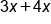To simplify, add 4x to 3x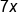Sum of 3x and 4x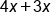To simplify, add 3x to 4xSum of 3x and 4x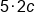To simplify, multiply 5 by 2c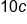Product of 5 and 2c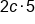To simplify, multiply 2c by 5Product of 2c and 5

In each example above, notice that the order in which applied the operation (either addition or multiplication) did not affect the solution. It is important to note that subtraction and division are not commutative.

big idea
Addition and multiplication is commutative. When adding terms, you can add them in any order you wish. When multiplying terms, you may multiply the terms in any order you wish.

# 2. Associative Properties of Addition and Multiplication

The associative property deals with how terms of an expression are grouped together. For algebraic expressions in which several terms are being added together, you can group terms together in any way in order to make simplification easier. Here is an example of the associative property of addition: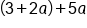We can group terms in any way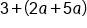Add 2a to 5a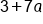Our Solution

In some cases, such as the example above, the associative property is helpful when grouping like terms together. We used the associative property first add 2a and 5a to get 7a, then we added 3 at the end.

The associative property holds true for multiplication as well, and works in a similar way to addition.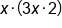We can group terms in any way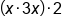Multiply x and 3x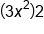Multiply by 2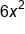Our Solution

# 3. Distributive Property

Often as we work with problems, there will be a set of parentheses that make solving a problem difficult, if not impossible. To get rid of these unwanted parentheses, we can use the distributive property. Using this property, we multiply the number in front of the parentheses by each term inside. Here are some examples: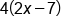Multiply each term by 4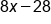Our Solution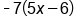Multiply each term by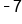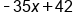Our Solution

hint
Notice that in the previous example, we multiplied each term inside the parentheses by a negative number. With the subtraction inside, this means we multiplied -7 by -6, to result in positive 42. The most common error in distributing is a sign error. Be careful with your signs!

summary
The commutative, associative, distributive properties, as well as factoring, can be extended to expressions that are involving variables. These properties will be useful when simplifying expressions and solving equations.

Source: Adapted from "Beginning and Intermediate Algebra" by Tyler Wallace, an open source textbook available at: http://wallace.ccfaculty.org/book/book.html

Rating